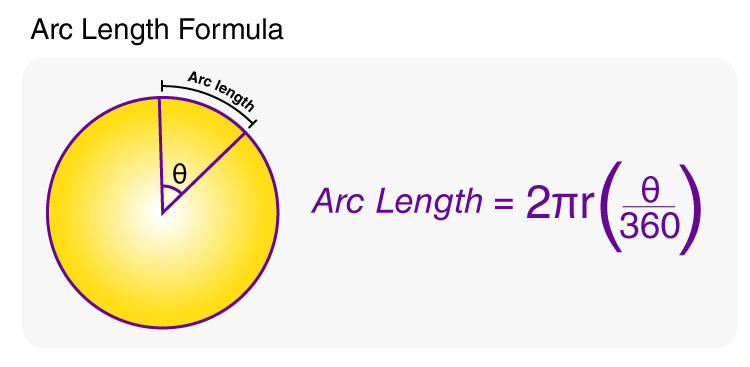# Arc Length Formula

Arc length formula is used to calculate the measure of the distance along the curved line making up the arc (a segment of a circle). In simple words, the distance that runs through the curved line of the circle making up the arc is known as the arc length. It should be noted that the arc length is longer than the straight line distance between its endpoints.Formula to Calculate Arc Length

## Formulas for Arc Length

The formula to measure the length of the arc is –

 Arc Length Formula (if θ is in degrees) s = 2 π r (θ/360°) Arc Length Formula (if θ is in radians) s = ϴ × r Arc Length Formula in Integral Form s= $$\int^{b}_a\sqrt{1+(\frac{dy}{dx})^2}dx$$

### Denotations in the Arc Length Formula

• s is the arc length
• r is the radius of the circle
• θ is the central angle of the arc

### Example Questions Using the Formula for Arc Length

Question 1: Calculate the length of an arc if the radius of an arc is 8 cm and the central angle is 40°.

Solution:

Central angle, θ = 40°

Arc length = 2 π r ×  (θ/360°)

So, s = 2 × π × 8 × (40°/360°)

= 5.582 cm

Question 2: What is the arc length for a function f(x) = 6 between x = 4 and x = 6?

Solution:

Since the function is a constant, the differential of it will be 0. So, the arc length will now be-

$$s=\int^{6}_4\sqrt{1 + (0)^2}dx$$

So, arc length (s) = (6 – 4) = 2.

### Practice Questions Based on Arc Length Formula

1. What would be the length of the arc formed by 75° of a circle having the diameter of 18 cm?
2. The length of an arc formed by 60° of a circle of radius “r” is 8.37 cm. Find the radius (r) of that circle.
3. Calculate the perimeter of a semicircle of radius 1. cm using the arc length formula.

### Also Check:

Stay tuned with BYJU’S to get more such mathematics related formulas and their explanations. Also, get various maths lessons, preparation tips, question papers, sample papers, syllabus, and other study materials by registering at BYJU’S.